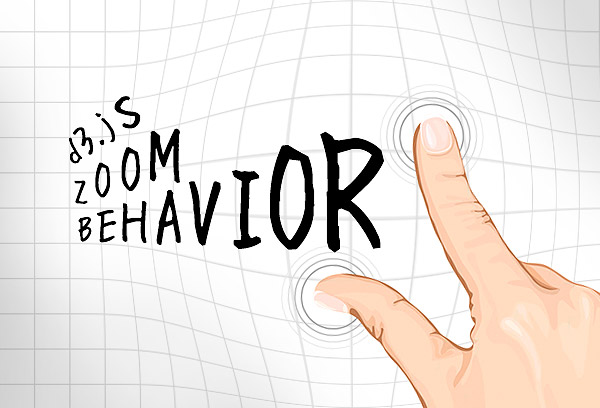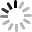# SVG D3.js - 縮放行為 ( Zoom Behavior )zoom 的用法和 drag 滿類似的，都是一開始要先用`d3.behavior.zoom();`宣告行為，然後再使用`call`來呼叫，不過 zoom 相對又稍微複雜了些，因為又多了一些參數需要設定，讓我們先來看一下它提供了哪些 api：

• zoom.translate([translate])：移動距離，預設為(0,0)
• zoom.scale([scale])：縮放大小比例，預設為(1)
• zoom.scaleExtent([extent])：縮放最大值與最小值，預設為(1,無限大)
• zoom.center([center])：預設值為 null，縮放中心點，會以滑鼠指標位置為中心點
• zoom.x([x])：預設值為 null，指定縮放時，應自動調整其域 x 縮放比例
• zoom.y([y])：預設值為 null，指定縮放時，應自動調整其域 y 縮放比例
• zoom.on(type, listener)：提供「zoomstart」、「zoom」和「zoomend」的監聽事件
• zoom.event(selection)：執行當下事件

``````var w = 800;
var h = 400;

var svg = d3.select('body')
.append('svg')
.attr({
'width':'100%',
'height':'100%'
}).style({
'border':'1px solid #000'
});
``````

``````var container = svg.append('g');

container.append('g')
.selectAll('line')
.data(d3.range(0, w, 30))
.enter()
.append('line')
.attr({
'x1': function(d) { return d; },
'y1': 0,
'x2': function(d) { return d; },
'y2': h,
'stroke':'#ddd',
'fill':'none'
});

container.append('g')
.selectAll('line')
.data(d3.range(0, h, 30))
.enter()
.append('line')
.attr({
'x1': 0,
'y1': function(d) { return d; },
'x2': w,
'y2': function(d) { return d; },
'stroke':'#ddd',
'fill':'none'
});
``````

``````var data = [
{'cx':150, 'cy':210, 'r':50, 'fill':'#ff0000'},
{'cx':75, 'cy':55, 'r':40, 'fill':'#00cc00'},
{'cx':200, 'cy':30, 'r':30, 'fill':'#0000ff'},
{'cx':650, 'cy':190, 'r':70, 'fill':'#0099cc'},
{'cx':300, 'cy':200, 'r':30, 'fill':'#ff9900'}
];

container.append("g").selectAll('circle')
.data(data)
.enter()
.append('circle')
.attr({
'cx': function(d){return d.cx;},
'cy': function(d){return d.cy;},
'r': function(d){return d.r;},
'fill': function(d){return d.fill;}
})
.style({
'cursor':'pointer'
});
````````````var x,y,s;

var zoom = d3.behavior.zoom()
.translate([0, 0])
.scaleExtent([1, 10])
.scale(1)
.on("zoom", zoomed);

function zoomed() {
x=d3.event.translate;
y=d3.event.translate;
s=d3.event.scale;
container.attr("transform", "translate(" + d3.event.translate + ") scale(" + d3.event.scale + ")");
}

var svg = d3.select('body')
.append('svg')
.attr({
'width':'100%',
'height':'100%'
}).style({
'border':'1px solid #000'
})
.call(zoom);
``````

``````d3.select('#reset').on('click',function(){
d3.transition().duration(250).tween("zoom", function() {
var si = d3.interpolate(s, 1);
var xi = d3.interpolate(x, 0);
var yi = d3.interpolate(y, 0);
return function(t){
svg.call(zoom.translate([xi(t),yi(t)]).scale(si(t)).event);
}
});
});
``````

``````d3.selectAll('circle').on('click',function(){
var circle = d3.select(this);
circle.transition().duration(250).tween("zoom", function() {
var r = this.getAttribute('r')*1;
var cx = this.getAttribute('cx')*1;
var cy = this.getAttribute('cy')*1;
var si = d3.interpolate(s, 4);
var xi = d3.interpolate(x, (w-r)/2-4*cx);
var yi = d3.interpolate(y, (h-r)/2-4*cy);
return function(t){
svg.call(zoom.translate([xi(t),yi(t)]).scale(si(t)).event);
}
});
});
``````